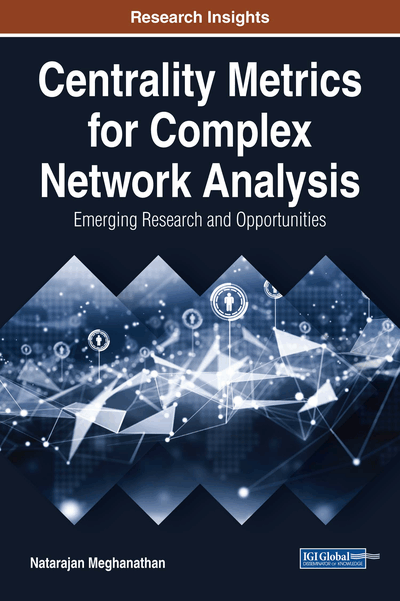# Centrality Metrics, Measures, and Real-World Network Graphs: Node and Edge Centrality Metrics, Correlation Measures, and Real-World Network Graphs

Copyright: © 2018 |Pages: 33
DOI: 10.4018/978-1-5225-3802-8.ch001

## Abstract

This chapter provides an introduction to various node and edge centrality metrics that are studied throughout this book. The authors describe the procedure to compute these metrics and illustrate the same with an example. The node centrality metrics described are degree centrality (DEG), eigenvector centrality (EVC), betweenness centrality (BWC), closeness centrality (CLC), and the local clustering coefficient complement-based degree centrality (LCC'DC). The edge centrality metrics described are edge betweenness centrality (EBWC) and neighborhood overlap (NOVER). The authors then describe the three different correlation measures—Pearson's, Spearman's, and Kendall's measures—that are used in this book to analyze the correlation between any two centrality metrics. Finally, the authors provide a brief description of the 50 real-world network graphs that are studied in some of the chapters of this book.
Chapter Preview
Top

## 2. Node Centrality Metrics

In this section, we describe the notion behind the five node centrality metrics (degree centrality: DEG, eigenvector centrality: EVC, betweenness centrality: BWC, closeness centrality: CLC and local clustering coefficient complement-based degree centrality: LCC’DC), their computation procedures and their theoretical computational time complexities as well as illustrate their computation with a running example graph. While the EVC and LCC’DC values range from 0 to 1, there are no upper or lower bounds for the values of the DEG, BWC and CLC metrics. Table 1 presents an outline of the classification of the centrality metrics to the three categories (neighborhood-based, shortest path-based and hybrid: that encompasses the features of both neighborhood-based and shortest path-based metrics).

Table 1.
Classification of the centrality metrics
 Node Centrality Metric Neighborhood-Based Shortest Path-Based Hybrid Degree Centrality (DEG) X Eigenvector Centrality (EVC) X Betweenness Centrality (BWC) X Closeness Centrality (CLC) X Local Clustering Coefficient Complement-based Degree Centrality (LCC’DC) X

## Complete Chapter List

Search this Book:
Reset